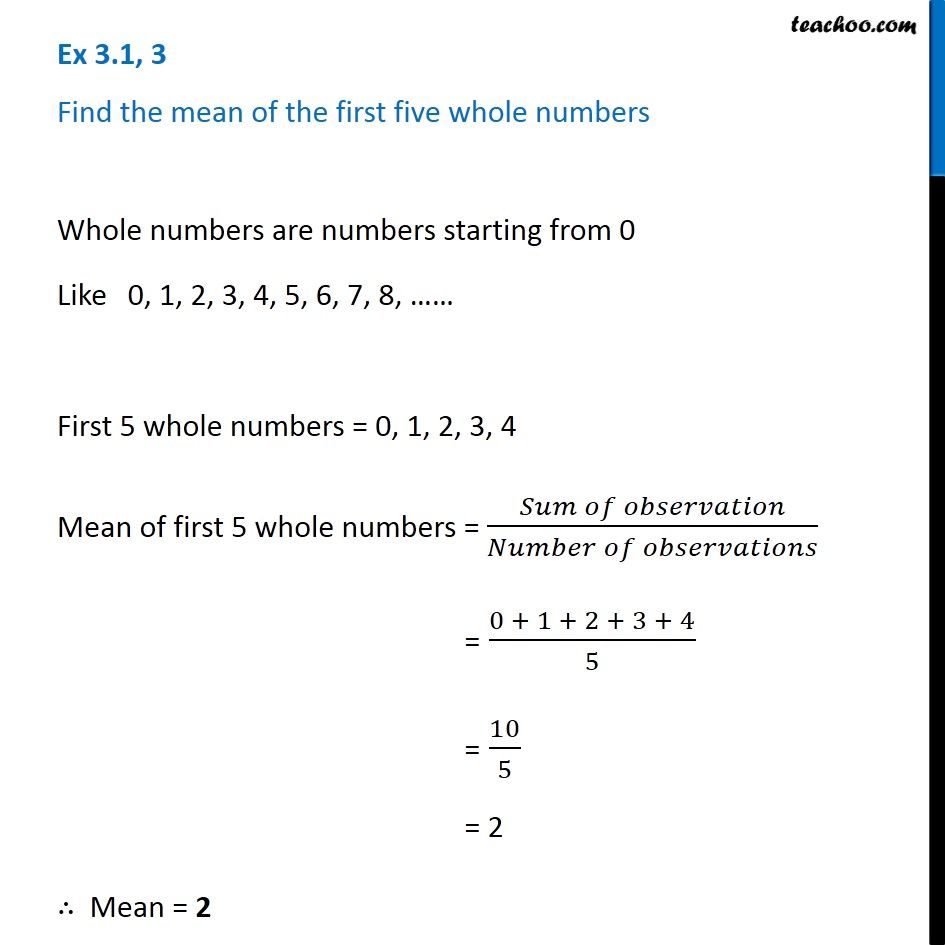1. Chapter 3 Class 7 Data Handling
2. Serial order wise
3. Ex 3.1

Transcript

Ex 3.1, 3 Find the mean of the first five whole numbers Whole numbers are numbers starting from 0 Like 0, 1, 2, 3, 4, 5, 6, 7, 8, …… First 5 whole numbers = 0, 1, 2, 3, 4 Mean of first 5 whole numbers = (𝑆𝑢𝑚 𝑜𝑓 𝑜𝑏𝑠𝑒𝑟𝑣𝑎𝑡𝑖𝑜𝑛)/(𝑁𝑢𝑚𝑏𝑒𝑟 𝑜𝑓 𝑜𝑏𝑠𝑒𝑟𝑣𝑎𝑡𝑖𝑜𝑛𝑠) = (0 + 1 + 2 + 3 + 4)/5 = 10/5 = 2 ∴ Mean = 2

Ex 3.1

Chapter 3 Class 7 Data Handling
Serial order wise